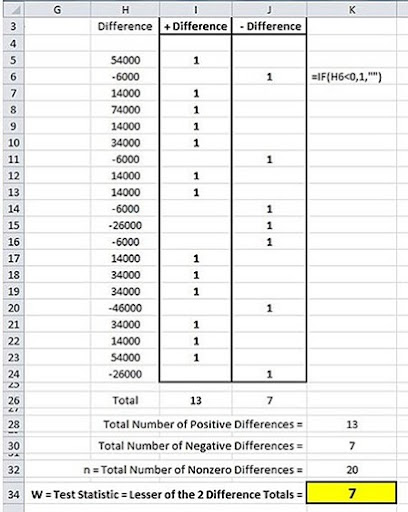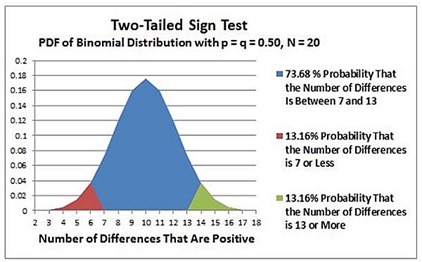## Saturday, May 31, 2014

### Sign Test As a 1-Sample t-Test Alternative in Excel 2010 and Excel 2013

This is one of the following six articles on 1-Sample t-Tests in Excel

1-Sample t-Test in 4 Steps in Excel 2010 and Excel 2013

Excel Normality Testing For the 1-Sample t-Test in Excel 2010 and Excel 2013

1-Sample t-Test – Effect Size in Excel 2010 and Excel 2013

1-Sample t-Test Power With G*Power Utility

Wilcoxon Signed-Rank Test in 8 Steps As a 1-Sample t-Test Alternative in Excel 2010 and Excel 2013

Sign Test As a 1-Sample t-Test Alternative in Excel 2010 and Excel 2013

# The Sign Test in Excel As an Alternative to the 1- Sample t-Test

The Sign Test along with the Wilcoxon One-Sample Signed-Rank Test are nonparametric alternatives to the one-sample t-Test when the normality of the sample or population cannot be verified and the sample size is small.

The Wilcoxon One-Sample Signed-Rank Test is significantly more powerful than the Sign Test but has a requirement of symmetrical distribution about a median for the difference sample data (the data set of the sample points minus the Constant of the Null Hypothesis). The Wilcoxon One-Sample Signed-Rank Test is based upon a normal approximation of its Test Statistic’s distribution. This requires that the difference sample be reasonably symmetrically distributed about a median.

The Sign Test has no requirements regarding the distribution of data but, as mentioned, is significantly less powerful than the Wilcoxon One-Sample Signed-Rank Test.

The Sign Test counts the number of positive and negative non-zero differences between sample data and the Constant from the Null Hypothesis in the one-sample t-Test. In this case that Constant = 186,000 because the Null Hypothesis of the one-tailed t-Test is as follows:

H0: x_bar = Constant = 186,000

This difference sample is calculated as follows:(Click On Image and See a larger Version)

A count of positive and negative differences in this sample is taken as follows:(Click On Image and See a larger Version)

The minimum count of positive or negative non-zero differences is designated as the Test Statistic W for this One-Sample Sign Test. Test Statistic W is named after Frank Wilcoxon who developed the test.

The objective of the two-tailed, one-sample t-Test was to determine whether to reject or fail to reject the Null Hypothesis that states that the mean monthly sales of retails stores in the one region is equal to the national average which is 186,000.

If the region’s mean store sales is equal to 186,000, then the probability of the monthly sales of any store in the region minus 186,000 being positive (greater than zero) is the same as the probability of being negative (less than zero). This probability is 50 percent.

Without knowing whether positive outcomes or negative outcomes are being counted, the probability of the mean monthly sales of the region’s stores being 186,000 is equal to the probability of a positive outcome (p) being 50 percent OR the probability of a negative outcome (q) being 50 percent.

The Null Hypothesis for this two-tailed, one-sample Sign Test states that the probability of a difference being positive (p) OR the probability of a difference being negative (q) is 50 percent. This can be expressed as follows:

H0: p=0.5 OR q=0.5

which would be expressed as follows:

H0: p=0.5 ∩ q=0.5

The Alternative Hypothesis would state the following:

H1: p≠0.5 ∩ q≠0.5

Each non-zero difference is classified as either positive or negative. This is a binary event because the classification of each difference has only two possible outcomes: the non-zero difference is either positive or negative.

The distribution of the outcomes of this binary event can be described by the binomial distribution as long as the following two conditions exist:

1) Each binary trial is independent.

2) The data from which the differences are derived are at least ordinal. The data can be ratio, interval, ordinal, but not nominal. The differences of “less than” and “greater than” must be meaningful even if the amount of difference is not, as would be the case with ordinal data but not with nominal data.

3) Each binary trial has the same probability of a positive outcome.

All of these conditions are met because of the following:

1) Each sample taken is independent of any other sample.

2) The differences are derived from continuous (either ratio or interval) data.

3) The proportion of positive differences versus negative differences is assumed to be constant in the population from which the sample of differences was derived.

The counts of the positive and negative differences both follow the binomial distribution. The binary event to be analyzed will be one of the two, i.e., either the count of positive differences OR the count of the negative differences. The conservative choice will be made by selecting the count that has the lowest number.

This count, whether it is the count of positive differences or the count of negative differences, is designated as W, the Test Statistic. This Test Statistic follows the binomial distribution because W represents the count of positive or negative outcomes of independent binary events that all have the same probability of a positive outcome.

As stated, the Null Hypothesis of this two-tailed, one-sample Sign Test is the following:

H0: p=0.5 ∩ q=0.5

The Null Hypothesis would be rejected if the p Value calculated from this test is less than alpha, which is customarily set at 0.05.

The logical operator OR represents the intersection of sets. The probability of Event A OR Event B occurring equals the sums of the probabilities of each occurring individually.

Pr(A ∩ B) = PR(A) + Pr(B)

The p Value of this test represents the probability that p = 0.5 given that the count of positive differences is less than or equal to W OR q = 0.5 given that the count of negative differences is less than or equal to W. Test Statistic W can represent either the count of positive OR negative differences and is set to the difference type that has the lower count.

The p value equals the probability that p = 0.5 if W equals UP TO the count of positive differences OR the probability that q = 0.5 if W equals UP TO the count of negative differences.

This p Value is expressed as follows:

p Value =

Pr (p = No. of Positive Differences ≤ W |p=0.5,n = No. of Non-Zero Differences)

Pr (q = No. of Negative Differences ≤ W |p=0.5,n = No. of Non-Zero Differences)

Since Pr(A ∩ B) = PR(A) + Pr(B)

p Value =

Pr (p = No. of Positive Differences ≤ W |p=0.5,n = 20 = No. of Non-Zero Differences)

+

Pr (q = No. of Negative Differences ≤ W |p=0.5,n =20 = No. of Non-Zero Differences)

Given that variable x is binomially distributed, the CDF (Cumulative Distribution Function) of the x ≤ X is calculated in Excel as follows:

F(X;n,p) = BINOM.DIST(X, n, p, 1)

This calculates the probability that up to X number of positive outcomes will occur in n total binary trials if the probability of a positive outcome is p for every trial. “1” specifies that the Excel formula will calculate the CDF and not the PDF.

Therefore the following can be calculated:

Pr (p = No. of Positive Differences ≤ W |p=0.5, n = Total No. of Non-Zero Differences) =

= BINOM.DIST(W, n, p,1)

= BINOM.DIST(7,20,0.5,1) = 0.1316

Pr (q = No. of Negative Differences ≤ W |p=0.5,n =20 = No. of Non-Zero Differences)

= 1 - BINOM.DIST(n - W, n, q,1)

= 1 - BINOM.DIST(13,20,0.5,1) = 0.1316

Due the symmetry of the binominal distribution, the following is true:

BINOM.DIST(W, n, p,1) = 1 - BINOM.DIST(n - W, n, q,1)

p Value = BINOM.DIST(W, n, p,1) + [1 - BINOM.DIST(n - W, n, q,1)]

p Value = 2 * BINOM.DIST(W, n, p,1)

p Value = 2 * BINOM.DIST(7,20,0.5,1) = 2 * 0.1316 = 0.2712

This is shown in the following Excel-generated graph of the PDF of the binomial distribution for this sign test. The parameters of this binomial distribution are Total Trials = N = 20 and the Probability of a Positive Outcome of Each Trial, p, equal 0.5. The Probability of a Negative Outcome, q, also equals 0.5.(Click On Image and See a larger Version)

This total p value (0.2712 = 0.1356 + 0.1356) is larger than alpha (set at 0.05). The Null Hypothesis is therefore not rejected at this alpha level. The Null Hypothesis for this test can be interpreted to state that the mean difference is equal to zero. This would be equivalent to stating that the mean monthly retails sales for the region is equal to the national average which is 186,000.

This example demonstrates how much less powerful the one-sample Sign Test is than the one-sample t-Test or the one-sample Wilcoxon Signed-Rank Test. The Sign Test did not come close to detecting a difference at the same alpha level that the other two tests performed in the previous blog articles did.

Excel Master Series Blog Directory

Statistical Topics and Articles In Each Topic

• Histograms in Excel
• Bar Chart in Excel
• Combinations & Permutations in Excel
• Normal Distribution in Excel
• t-Distribution in Excel
• Binomial Distribution in Excel
• z-Tests in Excel
• t-Tests in Excel
• Hypothesis Tests of Proportion in Excel
• Chi-Square Independence Tests in Excel
• Chi-Square Goodness-Of-Fit Tests in Excel
• F Tests in Excel
• Correlation in Excel
• Pearson Correlation in Excel
• Spearman Correlation in Excel
• Confidence Intervals in Excel
• Simple Linear Regression in Excel
• Multiple Linear Regression in Excel
• Logistic Regression in Excel
• Single-Factor ANOVA in Excel
• Two-Factor ANOVA With Replication in Excel
• Two-Factor ANOVA Without Replication in Excel
• Randomized Block Design ANOVA in Excel
• Repeated-Measures ANOVA in Excel
• ANCOVA in Excel
• Normality Testing in Excel
• Nonparametric Testing in Excel
• Post Hoc Testing in Excel
• Creating Interactive Graphs of Statistical Distributions in Excel
• Solving Problems With Other Distributions in Excel
• Optimization With Excel Solver
• Chi-Square Population Variance Test in Excel
• Analyzing Data With Pivot Tables and Pivot Charts
• SEO Functions in Excel
• Time Series Analysis in Excel
• VLOOKUP
• Simplifying Useful Excel Functions

#### 1 comment:

1.Now during the final exams, I have a lot of homework. I didn't have time to do everything on time, and my grades began to deteriorate. That's why I decided to look for helpers who could help me. I entrusted some of the tasks to create presentations to https://slidepeak.com/blog/what-does-corporate-presentation-template-mean-for-your-business-brand service and made the right choice. I received 2 of my presentations on time while doing other tasks.